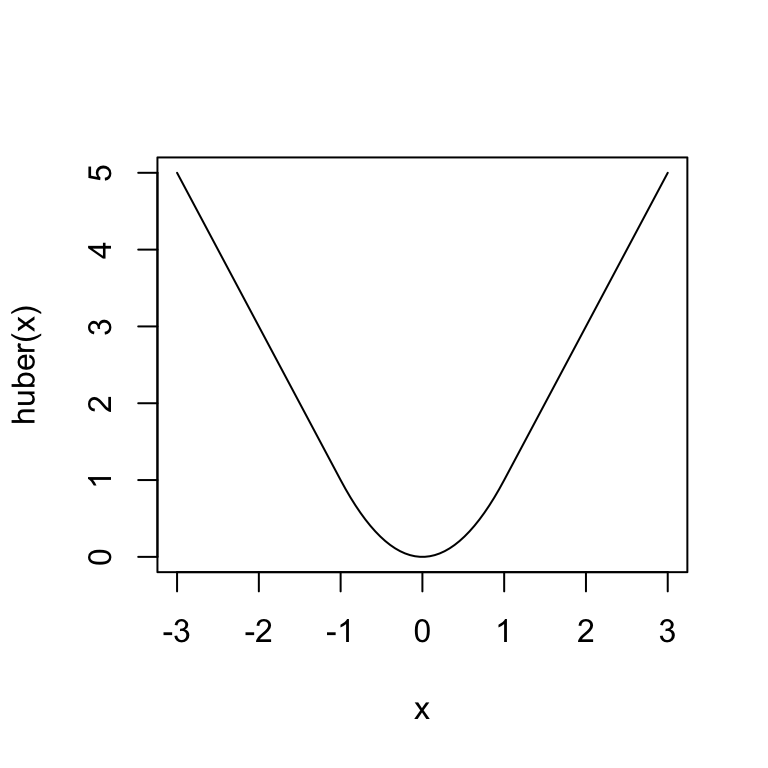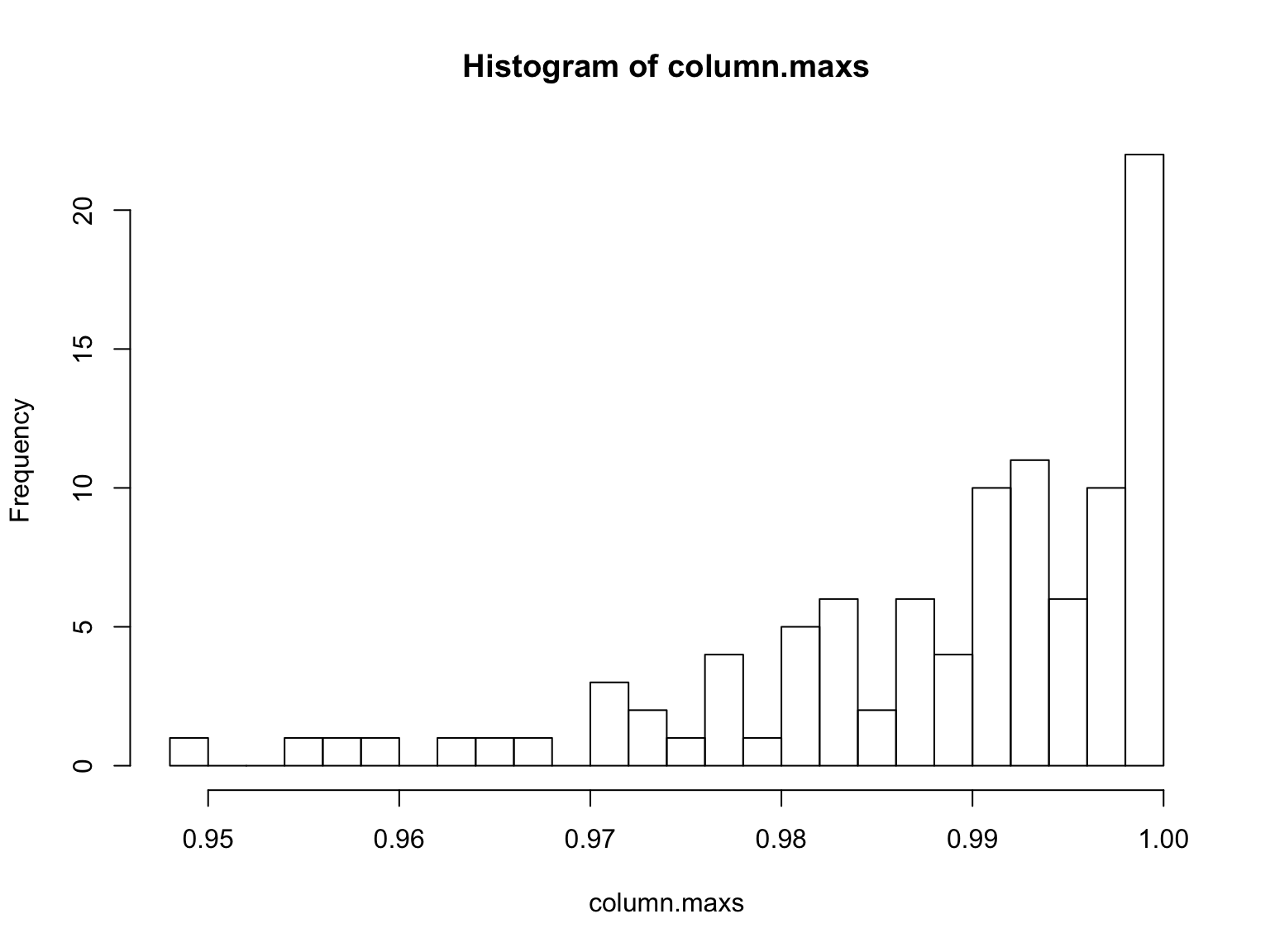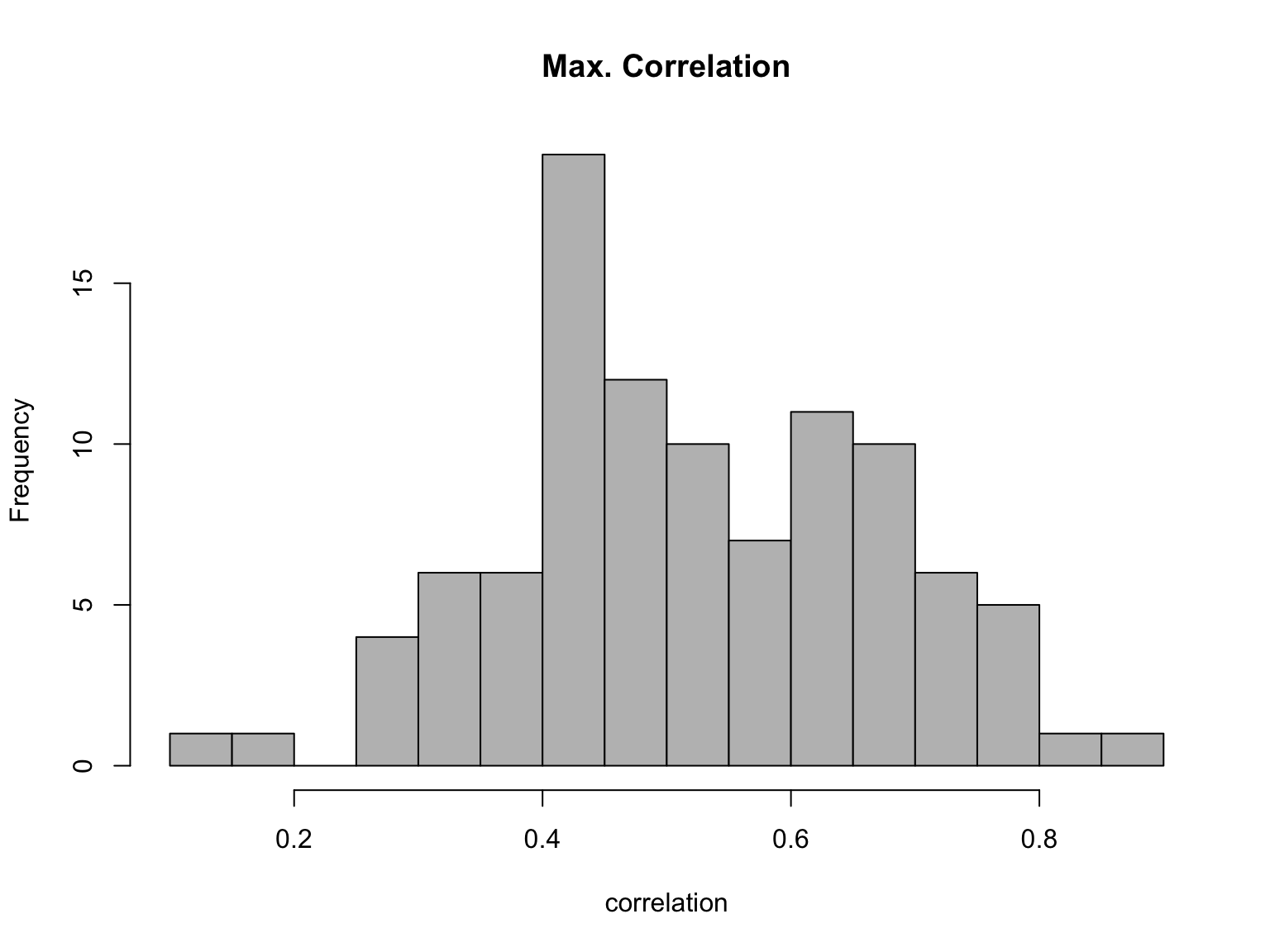# Minimal Advice on Programming in R

29 January 2019 (Lecture 5)

library(knitr)
opts_chunk\$set(size="small", cache=TRUE,
autodep=TRUE, warnings=TRUE, error=TRUE)

# Why do you need to do programming?

• Programs for data analysis are tools or machines
• Understand your tools!
• If you know how to make new tools, you always have the right tools
• Don’t distort your problem to match old tools
• Programming is making those tools
• Programming is also making your ideas clear

# Agenda for today

1. Style
2. Functions
3. Debugging
4. Avoiding iteration

# Style: Why?

• Code is about telling the computer what to do
• Code is also about communicating with other people
• Including communicating with yourself, later

# Style: Commandments

• Use meaningful names for variables, data-sets, functions, etc.
• Avoid magic numbers
• Indent your code blocks
• Comment your code
• Comment everything that seems clever or tricky
• Comment every trick you took from somewhere else
• Comment every function with purpose, inputs, outputs

# Functions

• Use functions to tie together related commands so they can be repeated
• Less work (in the long run)
• Fewer errors (get it right once, make improvements once)
• Clearer: allows for abstraction
• Lets you build complicated procedures out of modular, re-usable parts

# Functions: Example with Pareto distribution

• Distribution of wealth has very, very heavy tails
• A good (but not perfect) model is the Pareto distribution, pdf $f(x;\alpha,x_0) = \begin{cases} \frac{\alpha-1}{x_0}\left(\frac{x}{x_0}\right)^{-\alpha} & x \ge x_0\\ 0 & x < x_0 \end{cases}$
• We often want the quantiles (where does the 99% begin? the 99.9%? the 80%?)
• The mathematical quantile function is $Q(p; \alpha,x_0) = x_0(1-p)^{-\frac{1}{\alpha-1}}$
• Could do stuff like
# alpha = 2.33, x_0 = 6e8, p = 0.5
6e8 * (1-0.5)^(-1/(2.33-1))
##  1010391288
# alpha = 2.33, x_0 = 6e8, p = 0.4
6e8 * (1-0.4)^(-1/(2.33-1))
##  880957225
# alpha = 2.5, x_0 = 1e6, p = 0.92
1e6 * (1-0.92)^(-1/(2.5-1))
##  5386087

# Functions: Pareto example

• Bundle up the repetitive code into one function:
# Calculate quantiles of the Pareto distribution
# Inputs: desired quantile (p)
# exponent of the distribution (exponent)
# lower threshold of the distribution (threshold)
# Outputs: the pth quantile
qpareto.1 <- function(p, exponent, threshold) {
q <- threshold*((1-p)^(-1/(exponent-1)))
return(q)
}
• Now we can say:
qpareto.1(p=0.5, exponent=2.33, threshold=6e8)
##  1010391288
qpareto.1(p=0.4, exponent=2.33, threshold=6e8)
##  880957225
qpareto.1(p=0.92, exponent=2.5, threshold=1e6)
##  5386087

# Parts of a function

• Arguments: what does the function need to know?
• Return value: what does the function give back to R?
• Try to be explicit about what you return
• Use c() or list() to return more than one thing (We will see some examples of this)
• The body: do these things to the arguments to produce the return value

# Parts of a function: Pareto quantiles cont’d

# Calculate quantiles of the Pareto distribution
# Inputs: desired quantile (p)
# exponent of the distribution (exponent)
# lower threshold of the distribution (threshold)
# flag for whether to give lower or upper quantiles (lower.tail)
# Outputs: the pth quantile
qpareto.2 <- function(p, exponent, threshold, lower.tail=TRUE) {
if(lower.tail==FALSE) {
p <- 1-p
}
q <- threshold*((1-p)^(-1/(exponent-1)))
return(q)
}
qpareto.2(p=0.92, exponent=2.5, threshold=1e6)
##  5386087
qpareto.2(p=0.92, exponent=2.5, threshold=1e6, lower.tail=FALSE)
##  1057162

# When should we write functions?

• When we’re repeating the same thing multiple times
• Especially when: we’re repeating almost the same thing
• When we’re writing very similar code over and over
• When we’re breaking down a big job into smaller ones
• When we’re told to

# Chaining functions together

• Divide the big job (“come up with a confidence band”) into smaller parts
• Write a function for each part
• Make sure the outputs of the early steps can be used as inputs to the later steps
• Repeat (recurse) if need be: the sub-functions spawn sub-sub-functions, etc.
• We can often re-use the sub-functions

# Calling functions from other functions

• Functions can call other functions, including other functions we’ve written
• Could have avoided replicating the quantile code from qpareto.1 in qpareto.2:
# Calculate quantiles of the Pareto distribution
# Inputs: desired quantile (p)
# exponent of the distribution (exponent)
# lower threshold of the distribution (threshold)
# flag for whether to give lower or upper quantiles (lower.tail)
# Outputs: the pth quantile
qpareto.3 <- function(p, exponent, threshold, lower.tail=TRUE) {
if(lower.tail==FALSE) {
p <- 1-p
}
# Call qpareto.1() to do the actual calculation
q <- qpareto.1(p, exponent, threshold)
return(q)
}

# Functions calling other functions (cont’d)

• You will often be working with several intertwined functions at the same time
• The called functions have to be defined when they are used
• Example: the lecture code for cv.lm() calls a function, response.name(), to figure out what variable in a lm formula is the response
• response.name() is defined just after cv.lm(), but before the code +runs_ cv.lm()
• If you change (fix) the definition of a function, the new, revised one will be used thereafter (no snapshots)

# Debugging

• Eventually, you will break something.
• Problem: the final piece of code you write gives an incomprehensible error message
• Idea: Carefully characterize the bug, then narrow down where it could be until the the problem is obvious.
• Strategy: make sure all the lower-level pieces are working properly
• Start debugging from the bottom
• If all the small bits work on their own, the bug comes when you put them together

# Debugging: Test cases

• Use test cases
• “What should this do if it works?”
• “What should this not do if it’s broken?”
• When you meet a new bug, write a new test
• “How do I know if this is fixed?”
• Test could be very minimal: “It runs without crashing”

# Debugging: Some tools

• traceback(): Show all the calls leading to the last error
• Look for the last line of code you wrote
• This helps localize where things went wrong
• print(): Make R send output to the console
• Most useful a bit before where things crash/go crazy
• Remember to get rid of it when the bug is fixed!
• browser(): A convenient way to mess around inside a function
• When called within a function, gives you an R shell there
• Avoids problems with local variables disappearing
• A common trick: Executes [Dangerous Expression] and calls browser() if there is an error.
tryCatch({[Dangerous Expression]},warning=function(w){browser()},
error=function(e){browser()})

# Debugging: Pareto example continued

• I want to generate a Pareto random variable
• If $$F(x)$$ is the CDF for a distribution and $$U$$ is a uniform random variable, then $$F^{-1}(U)$$ has the $$F$$ distribution.
• New version of the quantile function that sanity-checks the arguments:
# Calculate quantiles of the Pareto distribution
# Inputs: desired quantile (p)
# exponent of the distribution (exponent)
# lower threshold of the distribution (threshold)
# flag for whether to give lower or upper quantiles (lower.tail)
# Outputs: the pth quantile
qpareto.4 <- function(p, exponent, threshold, lower.tail=TRUE) {
stopifnot(p >= 0, p <= 1, exponent > 1, threshold > 0)
q <- qpareto.3(p,exponent,threshold,lower.tail)
return(q)
}
• Try it with a bad input
qpareto.4(p=0.92,exponent=2.5,threshold=-1,lower.tail=FALSE)
## Error: threshold > 0 is not TRUE

# Debugging: Pareto example continued

• Now define a random-Pareto function. This version is (intentionally) buggy!
# Generate random numbers from the Pareto distribution
# Inputs: number of random draws (n)
# exponent of the distribution (exponent)
# lower threshold of the distribution (threshold)
# Outputs: vector of random numbers
rpareto <- function(n,exponent,threshold) {
x <- vector(length=n)
for (i in 1:n) {
x[i] <- qpareto.4(p=rnorm(1),exponent=exponent,threshold=threshold)
}
return(x)
}

# Debugging: Pareto example continued

• Our buggy code gives us errors!
rpareto(10)
## Error in qpareto.4(p = rnorm(1), exponent = exponent, threshold = threshold): argument "exponent" is missing, with no default
rpareto(n=10,exponent=2.5,threshold=1)
## Error: p >= 0 is not TRUE

# Debugging: The in-class exercise

EXERCISE: Where is the bug? Why doesn’t rpareto(n=10,exponent=2.5,threshold=1) work?

qpareto.1 <- function(p, exponent, threshold) {
q <- threshold*((1-p)^(-1/(exponent-1)))
return(q)
}

qpareto.3 <- function(p, exponent, threshold, lower.tail=TRUE) {
if(lower.tail==FALSE) {
p <- 1-p
}
q <- qpareto.1(p, exponent, threshold)
return(q)
}

qpareto.4 <- function(p, exponent, threshold, lower.tail=TRUE) {
stopifnot(p >= 0, p <= 1, exponent > 1, threshold > 0)
q <- qpareto.3(p,exponent,threshold,lower.tail)
return(q)
}

rpareto <- function(n,exponent,threshold) {
x <- vector(length=n)
for (i in 1:n) {
x[i] <- qpareto.4(p=rnorm(1),exponent=exponent,threshold=threshold)
}
return(x)
}

# Exercise: Solution

• We’ve checked the qpareto functions
• The error message is that p >= 0 is not true
• The error message comes from qpareto.4()
• So a negative value of p has to be going into qpareto.4() when it’s called by rpareto()
• And in fact that code says p=rnorm(1), and rnorm() draws from the standard normal, which is negative half the time
• That should be p=runif(1) (uniform distribution on $$[0,1]$$), not p=rnorm(1)
• As mentioned, this is the bug I actually made when I first wrote rpareto in 2004

# Avoid iteration

• Iterating in R is often quite slow
• Not (always) true in other languages
• R has lots of ways to avoid iteration
• This is often faster! And easier to follow!

# Avoid iteration: Pareto example cont’d

• A very iterative way of making many random Pareto draws:
# Generate random numbers from the Pareto distribution
# Inputs: number of random draws (n)
# exponent of the distribution (exponent)
# lower threshold of the distribution (threshold)
# Outputs: vector of random numbers
rpareto.1 <- function(n,exponent,threshold) {
x <- c()
for (i in 1:n) {
x <- c(x,qpareto.4(p=runif(1),exponent=exponent,threshold=threshold))
}
return(x)
}
system.time(rpareto.1(n=1e4,exponent=2.5,threshold=1))
##    user  system elapsed
##   0.323   0.018   0.343

# Avoid iteration: Pareto example cont’d

• A not-so-iterative way:
# Generate random numbers from the Pareto distribution
# Inputs: number of random draws (n)
# exponent of the distribution (exponent)
# lower threshold of the distribution (threshold)
# Outputs: vector of random numbers
rpareto.2 <- function(n,exponent,threshold) {
x = replicate(n, qpareto.4(p=runif(1),exponent=exponent,threshold=threshold))
return(x)
}

system.time(rpareto.2(n=1e4,exponent=2.5,threshold=1))
##    user  system elapsed
##   0.122   0.001   0.123

# Avoiding iteration: Vectorizing

• Lots of R functions are happy with vector arguments, and vectorize along them

• Our qpareto() functions can handle vector p arguments just fine:

qpareto.4(c(.1,.2,.3), 2.5, 1)
##  1.072766 1.160397 1.268434
• So use that:
# Generate random numbers from the Pareto distribution
# Inputs: number of random draws (n)
# exponent of the distribution (exponent)
# lower threshold of the distribution (threshold)
# Outputs: vector of random numbers
rpareto.3 <- function(n,exponent,threshold) {
x = qpareto.4(p=runif(n), exponent=exponent, threshold=threshold)
return(x)
}
system.time(rpareto.3(10000,exponent=2.5,threshold=1))
##    user  system elapsed
##   0.000   0.000   0.001

# Avoiding iteration: ifelse

• use ifelse to do conditional things to entire vectors
# Calculate Huber's loss function
# Input: vector of numbers x
# Return: x^2 for |x|<1, 2|x|-1 otherwise
huber <- function(x) {
return(ifelse(abs(x) <= 1, x^2, 2*abs(x)-1))
}
x = seq(from=-3,to=3,length.out=100)
plot(x,huber(x), type="l")# Avoiding iteration: matrix and vector operations

# Get some long vectors
n = 1e6
a = rnorm(n)
b = rnorm(n)
# Find the inner product with a for() loop
system.time({
total = 0
for(i in 1:length(a)){total = total + a[i]*b[i]}
})
##    user  system elapsed
##   0.058   0.000   0.058
# Find the inner product with matrix operations
system.time({
a %*% b
})
##    user  system elapsed
##   0.005   0.000   0.005

# Avoiding iteration: apply and friends

• Use apply to apply a function to rows or columns of a matrix
• Use sapply to apply a function to every element of a vector
#Make a 100x100 matrix of uniform [0,1] variables
X = matrix(runif(10000),nrow=100,ncol=100)
#Find the maximum of each column
column.maxs = apply(X, MARGIN = 2, FUN = max)
#Plot a histogram.  Do you know the distribution?
hist(column.maxs,20)• The tidyverse package defines a lot of new functions to push this idea to logical extremes

# Do not be afraid of iteration

• Some jobs are just inherently iterative
• Use it when that’s what you need to do
• If you’re storing stuff as you go, create the variables which you’re storing into outside the loop
sim.ar <- function(n,x1,phi=0,sd=1) {
x <- vector(length=n)
x <- x1
for (t in 2:n) {
x[t] <- phi*x[t-1]+rnorm(1,mean=0,sd=sd)
}
return(x)
}

# Returning vectors and lists

• A function can return one thing. Often you want to return more than one value:
• For several values of the same type, you can return a vector
• For several different sorts of values, you can return a list. You can even assign names!
• Returning a vector: Look at rpareto():
rpareto.3 <- function(n,exponent,threshold) {
x = qpareto.4(p=runif(n), exponent=exponent, threshold=threshold)
return(x) #If n>1, this is a vector!
}
• Returning a list: look at the end of cv_bws_npreg() from lecture 4:
  return(list(best.bw=best.bw,CV_MSEs=CV_MSEs,
fold_MSEs=fold_MSEs))

• Design from the top down
• Start from what you want to do (not how)
• Break that task into a few (2–6) smaller tasks
• Keep breaking tasks into smaller tasks until you can’t go further
• Code from the bottom up
• Write a function for each one, and test it
• Then write functions that call those functions (and test them)
• Keep going until you have top-level functions for the whole task

# What you need to remember

1. Use meaningful names and Comment your code
2. Write functions to bundle related commands together
3. Split big jobs into a bunch of related functions
4. Practice debugging
5. Avoid iteration, in favor of acting on whole objects

# Aside: Style: Things not to do with your main data frame

• Don’t use attach()
• Makes it really hard to track down errors
• Can shorten your code using with() instead
• Try not to call your main data set data
• vague
• the name of a function for loading data sets from packages
• often the name of arguments in model-fitting functions
• both of those make it hard to trace what’s going on
# Not what you want to read:
data.lm <- lm(growth~log(gdp)+underval, data=data)

# Aside: No-argument functions

• No-argument functions can be useful for simulation
# Function to return the biggest correlation of 5 length-10 Gaussian vectors
# Takes no arguments, so it can be used in a simulation
random.correlation.max = function(){
#Draw a 10x5 matrix of Gaussians
X = matrix(rnorm(50),nrow=10,ncol=5)
#Compute the correlation matrix
cor.matrix = cor(X)
#Find the biggest entry, making sure to avoid the diagonal (which is all 1)
return(max(cor.matrix[upper.tri(cor.matrix)]))
}
• Draw 100 samples and plot them
hist(replicate(100,random.correlation.max()),20,col='grey', main="Max. Correlation",xlab='correlation')# Aside: Functions refer to the current definition of other functions

• A very simple function that adds one to its argument
add.one = function(x){
x+1
}
• A function that calls add.one() to do its addition
add.stuff = function(x){
}
add.stuff(3)
##  4
• A terrible job rewriting add.one():
add.one = function(x){
}
add.stuff(3)
##  5